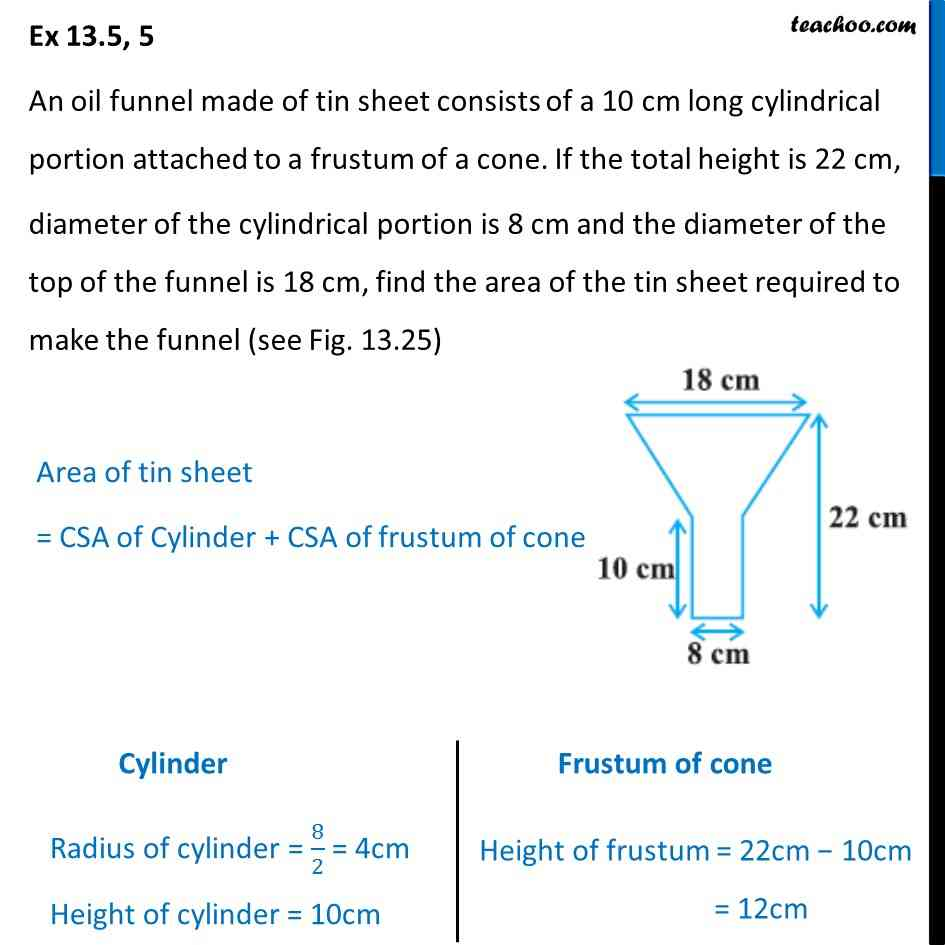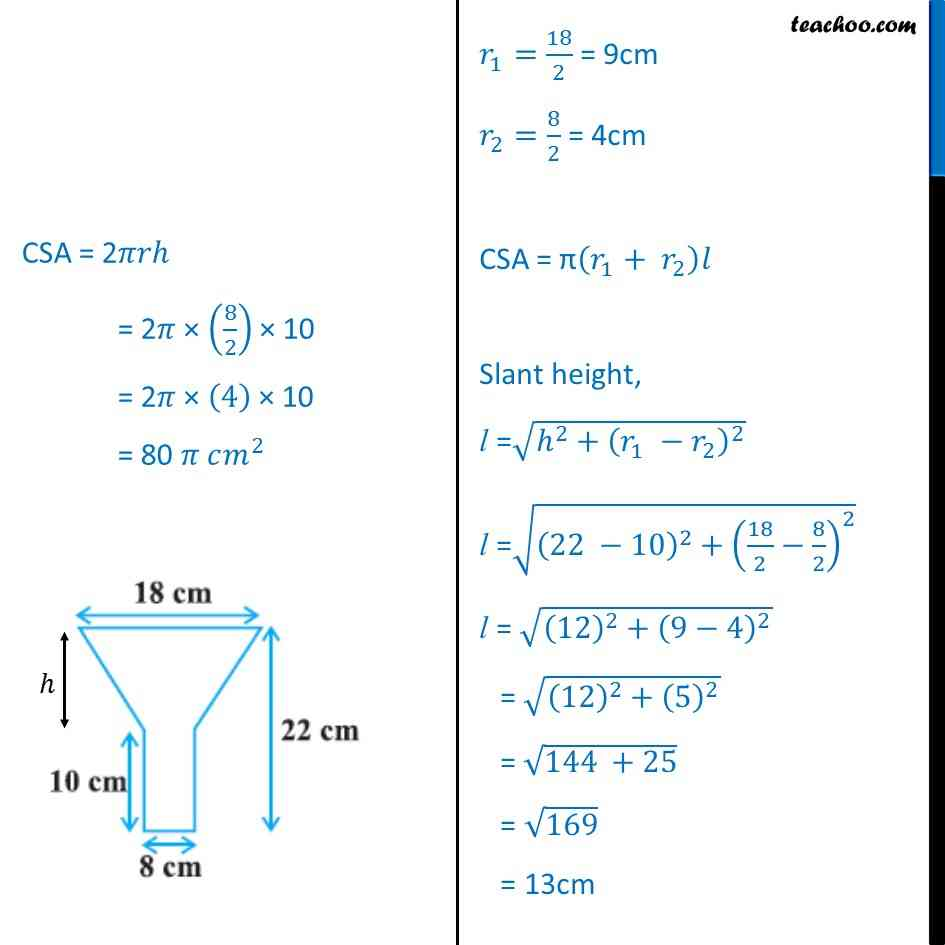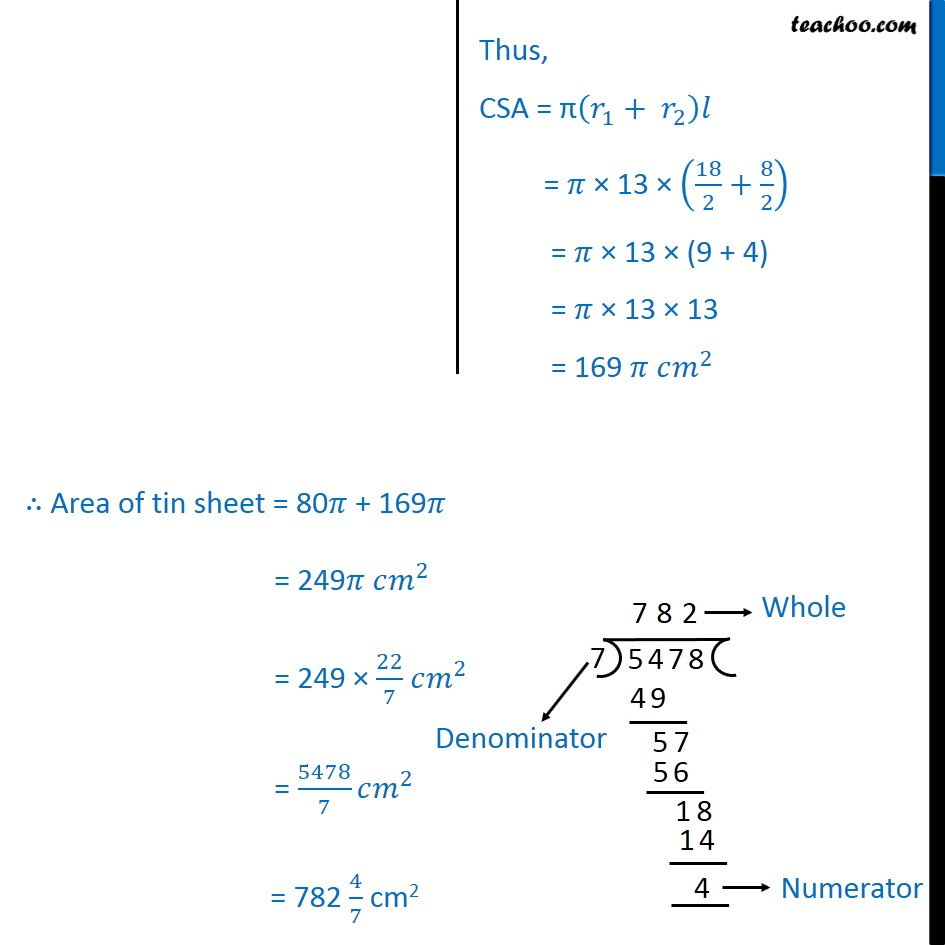Important Questions on Surface Area and Volume

Chapter 12 Class 10 Surface Areas and Volumes
Serial order wiseLearn in your speed, with individual attention - Teachoo Maths 1-on-1 Class

### Transcript

Question 5 An oil funnel made of tin sheet consists of a 10 cm long cylindrical portion attached to a frustum of a cone. If the total height is 22 cm, diameter of the cylindrical portion is 8 cm and the diameter of the top of the funnel is 18 cm, find the area of the tin sheet required to make the funnel (see Fig. 13.25) Area of tin sheet = CSA of Cylinder + CSA of frustum of cone Cylinder Radius of cylinder = 8/2 = 4cm Height of cylinder = 10cm CSA = 2 = 2 (8/2) 10 = 2 (4) 10 = 80 ^2 Frustum of cone Height of frustum = 22cm 10cm = 12cm _1=18/2 = 9cm _2=8/2 = 4cm CSA = ( _1+ _2 ) Slant height, l = ( ^2+( _1 _2 )^2 ) l = ((22 10)^2+(18/2 8/2)^2 ) l = ((12)^2+(9 4)^2 ) = ((12)^2+(5)^2 ) = (144 +25) = 169 = 13cm Thus, CSA = π(𝑟_1+ 𝑟_2 )𝑙 = 𝜋 × 13 × (18/2+8/2) = 𝜋 × 13 × (9 + 4) = 𝜋 × 13 × 13 = 169 𝜋 𝑐𝑚^2 ∴ Area of tin sheet = 80𝜋 + 169𝜋 = 249𝜋 𝑐𝑚^2 = 249 × 22/7 𝑐𝑚^2 = 5478/7 𝑐𝑚^2 = 782 4/7 cm2